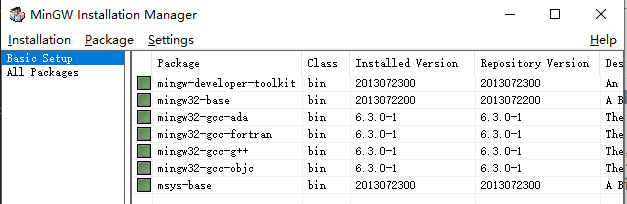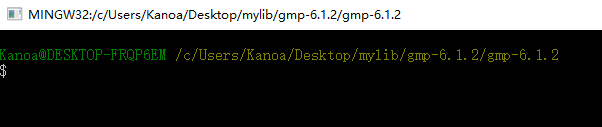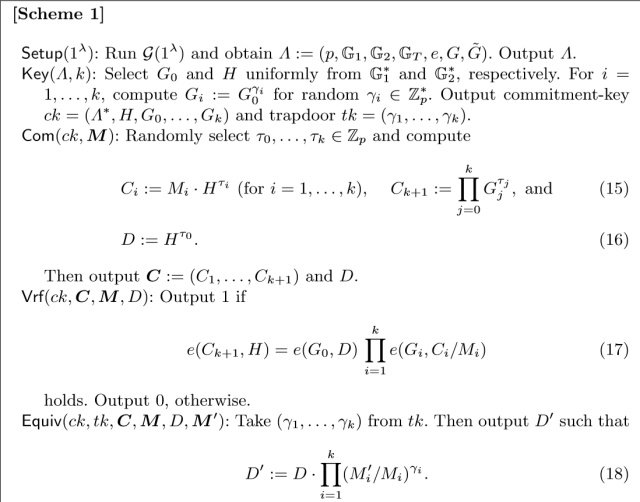# ubuntu make使用_双线性映射在密码学中的使用PBC库是一个Linux下的工程，如果想在win下运行，需要先将其编译成win下的lib库。pbc还要4.8版本的编译，后面好多的库都是依托Linux开发，放弃win下使用，转战Ubuntu。

Python 安装3.x，需要安装几个依赖 sudo apt-get install m4 flex bison libssl-dev

解压命令： tar -zxvf xx.tar.gz  tar -jxvf xx.tar.bz2

输入Python 进行命令行：测试下面代码
from charm.toolbox.integergroup import IntegerGroup

group1 = IntegerGroup()
group1.paramgen(1024)
g = group1.randomGen()
print(g)

vim ~/.bashrc  编辑当前用户环境变量文件

source ~/.bashrc  生效修改

from charm.toolbox.pairinggroup import PairingGroup,ZR,G1,G2,GT,pair
group2 = PairingGroup('SS512')
g1 = group2.random(G1)
g2 = group2.random(G2)
print('g1:', g1)
print('g2:', g2)from charm.toolbox.pairinggroup import PairingGroup,ZR,G1,G2,GT,pair
import random

# 初始化双线性群
BP = PairingGroup('SS512')

# 生成公共参数
g = BP.random(G1)
h = BP.random(G2)
p = 257
k = 100

# 生成陷门信息和公共参考串
ri = [random.randint(1, p) for _ in range(k)]
gi = [g ** each for each in ri]

# 生成消息
mi = [random.randint(1000, 2000) for _ in range(k)]

# 生成承诺信息
ti = [random.randint(1, p) for _ in range(k)]
t0 = random.randint(1, p)
ci = [mi[i] * (h ** ti[i]) for i in range(k)]
ck_1 = g ** t0
for j in range(k):
ck_1 = ck_1 * (gi[j] ** ti[j])
D = h ** t0

# 验证承诺
v1 = BP.pair_prod(ck_1, h)
v2 = BP.pair_prod(g, D)
for j in range(k):
v2 = v2 * BP.pair_prod(gi[j], ci[j]/mi[j])

if v1 == v2:
print('verify ok!')
print('v1:', v1)
print('v2:', v2)
08-3104-161万+
07-183万+
04-271万+
08-131万+
05-3040
01-1366
03-29648
03-011万+
03-21432
03-222707
11-25683
08-155180
04-266256
01-19780
04-171万+
04-095114
©️2020 CSDN 皮肤主题: 游动-白 设计师:白松林